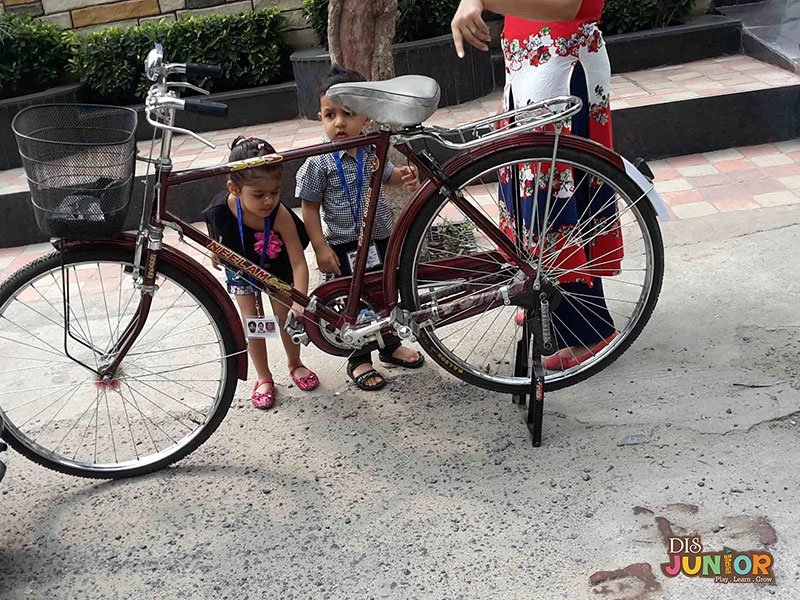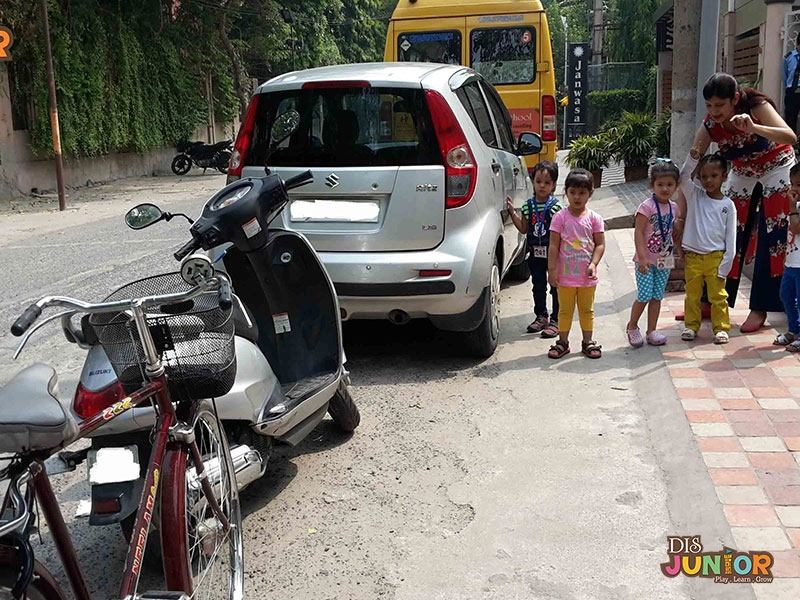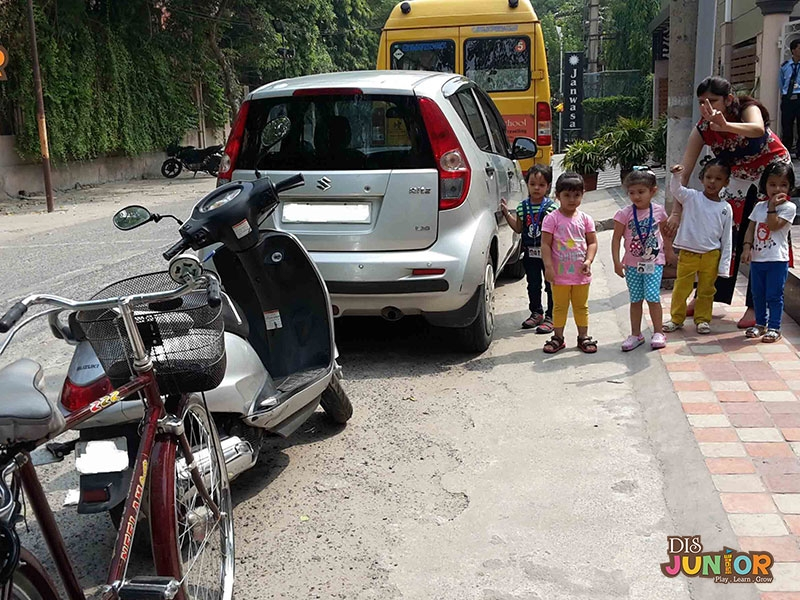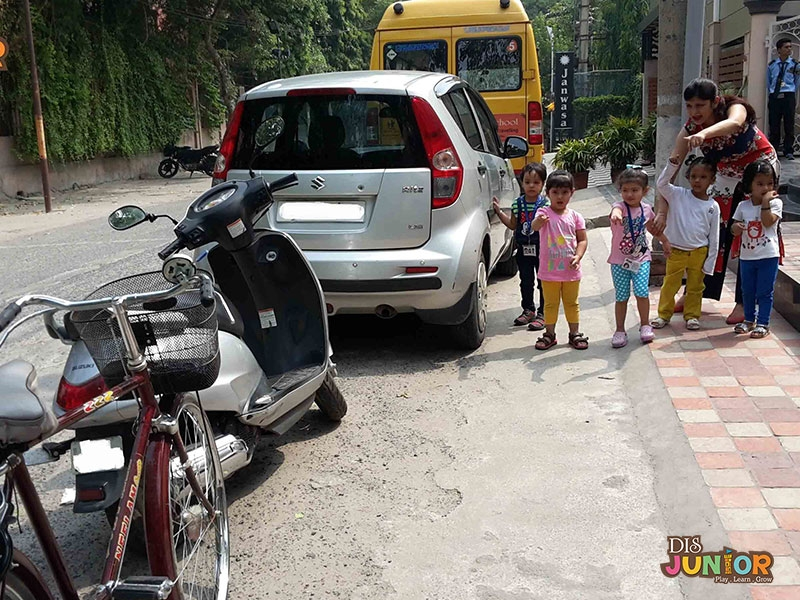## Life at DISIntroduction of Land TransportIntroduction of Land TransportIntroduction of Land TransportIntroduction of Land TransportIntroduction of Land TransportIntroduction of Land TransportIntroduction of Land TransportIntroduction of Land TransportIntroduction of Land TransportIntroduction of Land TransportIntroduction of Land TransportIntroduction of Land TransportIntroduction of Land TransportIntroduction of Land TransportIntroduction of Land TransportIntroduction of Land Transport